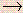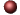Acids/Bases/Salts - Calculations(includes Balancing Redox)Examples ofMultiple Choice Questions

1.
How many grams of Ca(OH)2 are contained in 1500 mL of 0.0250 M Ca(OH)2 solution?
(a) 3.17 g
(b) 2.78 g
(c) 1.85 g
(d) 2.34 g
(e) 4.25 g

2.
What volume of 12.6 M HCl must be added to enough water to prepare 5.00 liters of 3.00 M HCl?
(a) 1.19 L
(b) 21.0 L
(c) 0.840 L
(d) 7.56 L
(e) 2.14 L

3.
What is the molarity of the salt produced in the reaction of 200 mL of 0.100 M HCl with 100 mL of 0.500 M KOH?
(a) 0.0325 M
(b) 0.0472 M
(c) 0.0667 M
(d) 0.0864 M
(e) 0.0935 M

4.
What volume of 0.50 M KOH would be required to neutralize completely 500 mL of 0.25 M H3PO4 solution?
(a) 2.5 x 102 mL
(b) 1.4 x 103 mL
(c) 83 mL
(d) 7.5 x 102 mL
(e) 5.2 x 102 mL

5.
A 0.6745 gram sample of KHP reacts with 41.75 mL of KOH solution for complete neutralization. What is the molarity of the KOH solution? (Molecular weight of KHP = 204 g/mol. KHP has one acidic hydrogen.)
(a) 0.158 M
(b) 0.099 M
(c) 0.139 M
(d) 0.079 M
(e) 0.061 M

6.
How many equivalents of phosphoric acid are contained in 300 mL of 4.00 M phosphoric acid? (Assume the acid is to be completely neutralized by a base.)
(a) 0.600 eq
(b) 1.20 eq
(c) 2.40 eq
(d) 3.60 eq
(e) 4.80 eq

7.
Calculate the normality of a solution that contains 4.5 g of (COOH)2 in 3000 mL of solution? (Assume the (COOH)2 is to be completely neutralized in an acid-base reaction.)
(a) 0.033 N
(b) 0.045 N
(c) 0.066 N
(d) 0.090 N
(e) 0.12 N

8.
What volume of 0.100 N HNO3 is required to neutralize 50.0 mL of a 0.150 N solution of Ba(OH)2?
(a) 50.0 mL
(b) 75.0 mL
(c) 100. mL
(d) 125 mL
(e) 150. mL

9.
How many grams of NaOH would be required to neutralize all the acid in 75.0 mL of 0.0900 N H2SO4?
(a) 0.540 g
(b) 0.270 g
(c) 1.32 g
(d) 0.660 g
(e) 0.859 g

10.
What is the oxidation number for carbon in CaC2O4?
(a) 0
(b) +2
(c) +3
(d) +4
(e) +6

11.
Balance the molecular equation for the following redox reaction. What is the sum of the coefficients? Don't forget coefficients of one. Use the smallest whole number coefficients possible.
H2SO4(aq) + HI(aq)I2(s) + SO2(g)
(a) 7
(b) 9
(c) 11
(d) 13
(e) 5

12.
For the reaction between permanganate ion and sufite ion in basic solution, the unbalanced equation is:
MnO4- + SO32-MnO2 + SO42-

When this equation is balanced using the smallest whole number coefficients possible, the number of OH- ions is
(a) two on the right.
(b) two on the left.
(c) three on the right.
(d) four on the right.
(e) four on the left.

13.
Balance the following redox equation in acidic solution with the smallest whole number coefficients possible. What is the sum of all the coefficients? (Do not forget coefficients of one.)
Cu + SO42-Cu2+ + SO2 (in acidic solution)
(a) 9
(b) 10
(c) 11
(d) 12
(e) 13

14.
When the following equation is balanced with the smallest possible set of integers, what is the sum of all the coefficients? (Do not forget coefficients of one.)
Cr2O72- + H2SCr3+ + S (in acidic solution)
(a) 13
(b) 24
(c) 19
(d) 7
(e) 29

15.
When the following equation is balanced with the smallest possible set of integers, what is the sum of all the coefficients? (Do not forget coefficients of one.)
MnO4- + Se2-MnO2 + Se (in basic solution)
(a) 20
(b) 22
(c) 24
(d) 26
(e) 28

16.
Consider the following unbalanced equation in acidic solution:
NaClO3 + H2O + I2HIO3 + NaCl
A 25.0 mL sample of 0.0833 M NaClO3 reacted with 30.0 mL of an aqueous solution of I2. How many grams of I2 were contained in the I2 solution?
(a) 0.264 g
(b) 0.397 g
(c) 0.236 g
(d) 0.159 g
(e) 0.317 g

17.
Consider the following unbalanced net ionic equation:
NO2- + MnO4-NO3- + Mn2+ (in acidic solution)
What is the molarity of a sodium nitrite, NaNO2, solution if 30.0 mL of it just reacts with 0.238 grams of KMnO4?
(a) 0.410 M
(b) 0.126 M
(c) 0.0502 M
(d) 0.251 M
(e) 0.0316 M

18.
What is the equivalent weight (in grams) of copper(II) nitrate for use in a reaction involving the conversion of copper(II) to copper metal?
(a) 46.9 g/eq
(b) 93.8 g/eq
(c) 187.6 g/eq
(d) 375.2 g/eq
(e) 562.8 g/eq

19.
What is the normality of a K2Cr2O7 solution prepared by dissolving 5.00 g of K2Cr2O7 in 200 mL of solution, which will be used in the following unbalanced reaction?
Cr2O72- + SO32-Cr3+ + SO42- (in acidic solution)
(a) 0.733 N
(b) 0.226 N
(c) 0.510 N
(d) 0.441 N
(e) 0.810 N

20.
What mass of KMnO4 must be dissolved to prepare 1.25 L of 0.110 N KMnO4 solution? It is used in the reaction in which MnO4- ions oxidize Fe2+ into Fe3+ ions and are reduced to Mn2+ ions under acidic conditions?
(a) 4.34 g
(b) 23.8 g
(c) 115 g
(d) 19.1 g
(e) 70.6 g

21.
A 0.250 M solution of Na2C2O4 is to be used in a reaction in which the C2O42- will be oxidized to CO2. What is the normality of this Na2C2O4 solution?
(a) 0.250 N
(b) 1.00 N
(c) 0.125 N
(d) 0.0625 N
(e) 0.500 N

22.
What volume of a 0.150 N KI solution is required to react in basic solution with 34.1 mL of a 0.216 N solution of KMnO4? The products in the reaction include MnO2 and IO3-.
(a) 25.4 mL
(b) 37.9 mL
(c) 12.6 mL
(d) 98.2 mL
(e) 49.1 mL

23.
Calculate the normality of a NaClO solution if 35.00 mL of the solution is required to react with 0.615 g of Zn according to the following unbalanced equation:
Zn + ClO-Zn(OH)2 + Cl- (in basic solution)
(a) 0.537 N
(b) 0.275 N
(c) 0.108 N
(d) 0.366 N
(e) 0.791 N

24.
A solution of nitrous acid was standardized in a reaction where HNO2NO3- and its concentration was determined to be 0.100 N nitrous acid. What volume of this 0.100 N nitrous acid solution would be required to oxidation of 0.200 g of CoCl2 to CoCl3 according to the following net ionic equation?
Co2+ + HNO2Co3+ + NO (in acidic solution)
(a) 33.9 mL
(b) 15.4 mL
(c) 7.70 mL
(d) 67.8 mL
(e) 30.8 mL

25.
What is the sum of all coefficients when the following net ionic equation is balanced using the smallest whole number coefficients possible? Do not forget coefficients of one.
MnO4- + Mn2+MnO2 (in basic solution)
(a) 19
(b) 16
(c) 13
(d) 11
(e) 7

1. (b) 2. (a) 3. (c) 4. (d) 5. (d) 6. (d) 7. (a) 8. (b) 9. (b) 10. (c) 11. (a) 12. (a) 13. (b) 14. (b) 15. (b) 16. (e) 17. (b) 18. (b) 19. (c) 20. (a) 21. (e) 22. (e) 23. (a) 24. (e) 25. (b)Click here to return to the top.Choose your next chapter:
Fundamentals of Chemistry | Chemical Formulas & Composition Stoichiometry | Chemical Equations & Rxn Stoichiometry | Types of Chemical Reactions |
| Atomic Structure | Chemical Periodicity | Chemical Bonding | Molecular Structure/Covalent Bonding Theories| Molecular Orbital Theory |
| Acids/Bases/Salts - Theory & Rxns | Acids/Bases/Salts - Calculations (including Balancing Redox) | Gases | Solids & Liquids | Solutions |
| Thermodynamics | Kinetics | Equilibrium | Aqueous Equilibrium - Acids/Bases/Salts | Aqueous Equilibrium - Buffers & Titrations |
| Aqueous Equilibrium - Slightly Soluble Salts | Electrochemistry | Metallurgy | Metal Properties & Rxns | Nonmetals & Metalloids |
| Coordination Compounds | Nuclear Chemistry | Organic Chem - Formulas/Names/Properties | Organic Chem - Shapes/Rxns/Biopolymers |To report any corrections, please e-mail Dr. Wendy Keeney-Kennicutt.